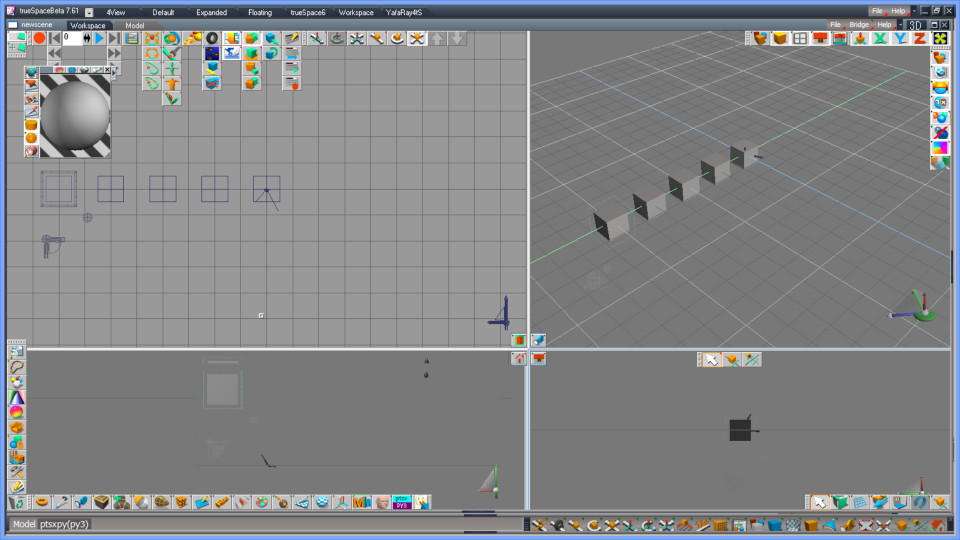## 0040. Tips for Python novice

Getting started, ptsxpy, Python language, trueSpace, programming, syntax highlighting for text editor, etc.
Note: it's provisional and topics will be added and revised at random till some sort of documentations are prepared.
3DfromNULL
Posts: 43
Joined: 2018-Jul-13 9:35

### 0040. Tips for Python novice

Comment

Code: Select all

# This whole line is comment, and ignored by Python interpreter.
import ptsxpy as p  # from the sharp sign to the new line is also comment
# But the sentence written before (i.e. left side of) the sharp sign is interpreted by Python.

Indentation
In a Python script, the indentation level (i.e. blank preceding to each line) is significant. The author uses 4 blank spaces for indentation in all sample scripts according to the official documents distributed by python.org: "PEP 8 -- Style Guide for Python Code" .

Code: Select all

#------------------------------
# Crete and move some cubes.
#------------------------------
import ptsxpy as p

x = 0.

for i in range( 5 ):
print( "i=", i )
cb1 = p.CreateCube( 2 , 1., 1., 1. )
loc = Vec3f( x, 0., 0. )
print( "loc=", loc.prt() )
p.GNodeSetLocation( cb1, loc.p )
x += 2.

p.SceneDraw()As show in example above, a for-loop does not have any parenthesis, have a colon ":" and indentation for all lines included in the loop instead. In case of nesting (another loop in the loop), we need to add 4 spaces to each line in the inner loop.

Types and Literals
Types which you minimally need to know for ptsxpy are;
• int ---- an integer value. e.g. -123
• float ---- a (64 bit) floating point value. e.g. 3.14
• string ---- a quoted string that consists of none or more characters. e.g. "abc"
• tuple ---- an object parenthesized by round brackets and it consists of none or more comma-separated elements. e.g. (123, "ab", 0.1 )
• list ---- an object parenthesized by square brackets and it consists of none or more comma-separated elements. e.g. [123, "ab", 0.1 ]
• dict ---- an object parenthesized by curly brackets and it consists of none or more comma-separated pair of key:value elements. e.g. {"a":123, "3":"B"}
List and dict are mutable, others of these are immutable.

Functions, mutable and immutable
In python, you can use functions in order to package specific sequences as subroutines as with other programming languages. To declare a function, write "def" followed by white blank(s), a function name, parameters in parenthesis, and colon ":". The code belonging to the function has one unit of indentation.

Code: Select all

def func1( a, b ):
print( "a=", a, ", b=", b )
a = a + 1
return a * b

def func2( lst1 ):
for i in range( 7, 10 ):  # i.e. loop for 7, 8, 9
lst1.append( i )   # add a value to a list as the last element

c = 5
d = 6
e = func1( c, d ) + func1( 2, 3 )   # assign (5+1)*6 + (2+1)*3 to a variable e
print( "c=", c, ", d=", d, ", e=", e )
l1 = []   # an empty list
func2( l1 )
print( "l1=", l1 )


Code: Select all

Executing [.... xxxxx.py]

a= 5 , b= 6
a= 2 , b= 3
c= 5 , d= 6 , e= 45
l1= [7, 8, 9]
.... xxxxx.py ended.----------

A function cannot change (rewrite) caller's immutable object like an integer, a tuple, etc. In the example above, the func1() can return a calculated value, but it cannot change main routine's immutable variables. In the example above, the variable c is unchanged after the func1() is called (though in case of C/C++, a function can change memory area pointed by parameters). A function can change elements of mutable object like list object or dict object. In the example above, l1 is a list, the func2() adds elements to it, and the l1's content is changed as a result.

The ptsxpy in its early versions (till about y2017) had a specification where it uses list objects for parameter to API functions, but the author changed so that user Python scripts directly uses C++ pointer using predefined classes (like Vec3f) because the older spec is bit difficult to understand and far apart from the tsxAPI's spec.